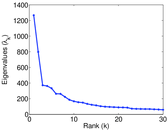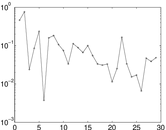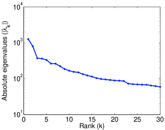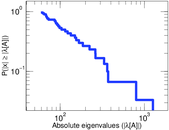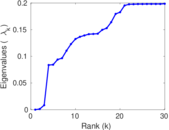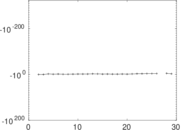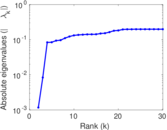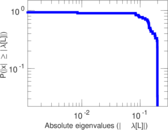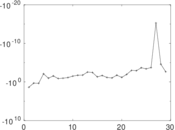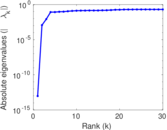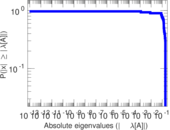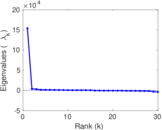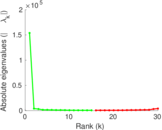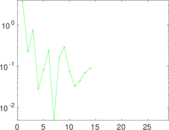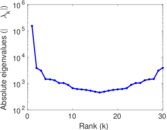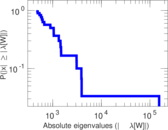# Wiktionary edits (fa)

This is the bipartite edit network of the Persian Wiktionary. It contains users and pages from the Persian Wiktionary, connected by edit events. Each edge represents an edit. The dataset includes the timestamp of each edit.

 Code `mfa` Internal name `edit-fawiktionary` Name Wiktionary edits (fa) Data source http://dumps.wikimedia.org/ AvailabilityDataset is available for download Consistency checkDataset passed all tests Category Authorship network Dataset timestamp 2017-10-20 Node meaning User, article Edge meaning Edit Network formatBipartite, undirected Edge typeUnweighted, multiple edges Temporal dataEdges are annotated with timestamps

## Statistics

 Size n = 153,841 Left size n1 = 1,485 Right size n2 = 152,356 Volume m = 735,491 Unique edge count m̿ = 343,141 Wedge count s = 7,080,337,446 Cross count x = 3,837,064,202,469,971,968 Square count q = 2,457,279,801 4-Tour count T4 = 47,980,332,650 Maximum degree dmax = 302,111 Maximum left degree d1max = 302,111 Maximum right degree d2max = 334 Average degree d = 9.561 70 Average left degree d1 = 495.280 Average right degree d2 = 4.827 45 Fill p = 0.001 516 65 Average edge multiplicity m̃ = 2.143 41 Size of LCC N = 152,426 Diameter δ = 12 50-Percentile effective diameter δ0.5 = 1.960 22 90-Percentile effective diameter δ0.9 = 3.995 09 Median distance δM = 2 Mean distance δm = 3.122 64 Gini coefficient G = 0.783 752 Balanced inequality ratio P = 0.199 519 Left balanced inequality ratio P1 = 0.023 699 8 Right balanced inequality ratio P2 = 0.279 631 Relative edge distribution entropy Her = 0.661 485 Tail power law exponent γt = 2.121 00 Tail power law exponent with p γ3 = 2.121 00 p-value p = 0.000 00 Left tail power law exponent with p γ3,1 = 1.431 00 Left p-value p1 = 0.227 000 Right tail power law exponent with p γ3,2 = 2.121 00 Right p-value p2 = 0.000 00 Degree assortativity ρ = −0.529 076 Degree assortativity p-value pρ = 0.000 00 Spectral norm α = 1,266.10 Algebraic connectivity a = 0.001 168 71 Controllability C = 150,399 Relative controllability Cr = 0.981 371

## Plots

### Fruchterman–Reingold graph drawing### Degree distribution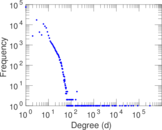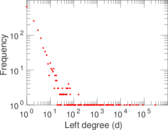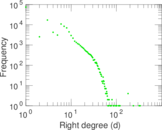### Cumulative degree distribution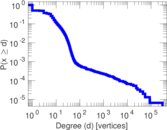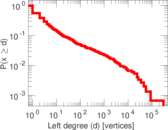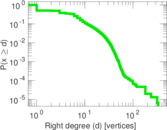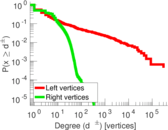### Lorenz curve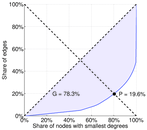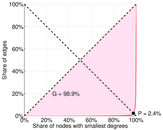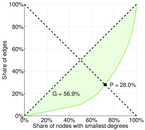### Spectral distribution of the adjacency matrix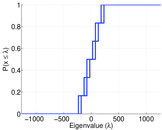### Spectral distribution of the normalized adjacency matrix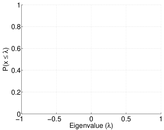### Spectral distribution of the Laplacian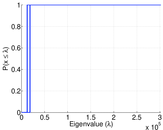### Spectral graph drawing based on the adjacency matrix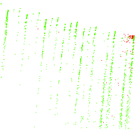### Spectral graph drawing based on the Laplacian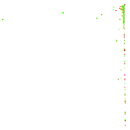### Spectral graph drawing based on the normalized adjacency matrix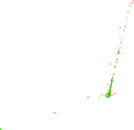### Degree assortativity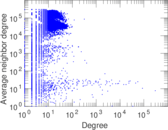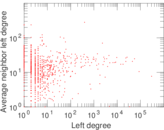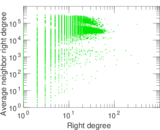### Zipf plot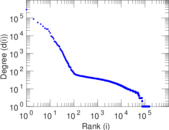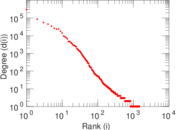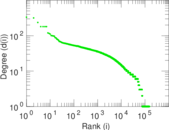### Hop distribution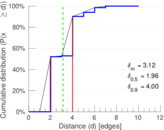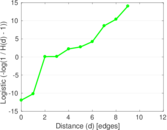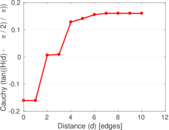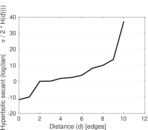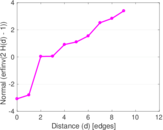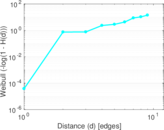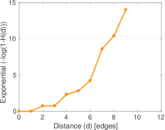### Delaunay graph drawing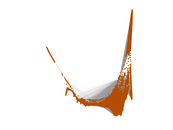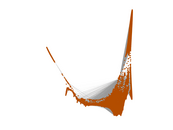### Edge weight/multiplicity distribution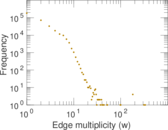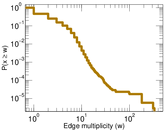### Temporal distribution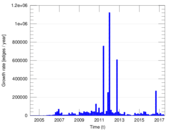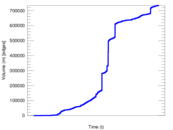### Diameter/density evolution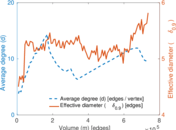### Matrix decompositions plots